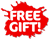Skip to content

# Texas Instruments MultiView Scientific Calculator - 34MV/TBL/1L1/A

Condition: New
Discontinued
Sold out
SKU TEX34MVTBL1L1A
\$34.99
\$34.99 - \$34.99
Current price \$34.99##### Item Description
The TI-34 MultiView scientific calculator was designed with educator input in mind for use in these middle grades math and science classesX

## Overview

View more calculations at a time

Four-line display allows you to enter more than one calculation; compare results and explore patterns; all on the same screen.

MathPrint feature

Use the MathPrint feature to display expressions; symbols; and fractions just as they appear in textbooks.

Explore fractions

Explore fraction simplification; integer division and constant operators.

Investigate patterns

Explore patterns via list conversions to see different number formats like decimal; fraction and percent side-by-side.

Product Features:

• Four-line display
• One- and two-variable statistics
• MathPrint feature
• Step-by-step fraction simplification
• Edit; cut; and paste entries
• Solar and battery powered
• Specifications:

Built-in functionality:

• Review and edit previous entries via a scrollable home screen
• Paste inputs or outputs into new calculations
• MathPrint mode for input in math notation; including π; square roots; fractions; percents and exponents plus math notation output for fractions
• CLASSIC mode for similar entry and compatibility with previous two-line scientific models like the TI-34II pixels
• π symbol on input; some calculations in terms of pi and pi to the maximum decimal places of the calculator
• Toggle key to change the form of answers between fraction and decimal; or between π terms and decimal
• Fraction/decimal/percent conversions
• Change between improper fractions and mixed numbers; plus a mode setting for mixed number or improper fraction output as the default
• Simplification of fractions feature to simplify a fraction one factor at a time instead of automatically simplifying to lowest terms

View more calculations at a time

Four-line display allows you to enter more than one calculation; compare results and explore patterns; all on the same screen.

MathPrint feature

Use the MathPrint feature to display expressions; symbols; and fractions just as they appear in textbooks.

Explore fractions

Explore fraction simplification; integer division and constant operators.

Investigate patterns

Explore patterns via list conversions to see different number formats like decimal; fraction and percent side-by-side.

Product Features:

• Four-line display
• One- and two-variable statistics
• MathPrint feature
• Step-by-step fraction simplification
• Edit; cut; and paste entries
• Solar and battery powered
• Specifications:

Built-in functionality:

• Review and edit previous entries via a scrollable home screen
• Paste inputs or outputs into new calculations
• MathPrint mode for input in math notation; including π; square roots; fractions; percents and exponents plus math notation output for fractions
• CLASSIC mode for similar entry and compatibility with previous two-line scientific models like the TI-34II pixels
• π symbol on input; some calculations in terms of pi and pi to the maximum decimal places of the calculator
• Toggle key to change the form of answers between fraction and decimal; or between π terms and decimal
• Fraction/decimal/percent conversions
• Change between improper fractions and mixed numbers; plus a mode setting for mixed number or improper fraction output as the default
• Simplification of fractions feature to simplify a fraction one factor at a time instead of automatically simplifying to lowest terms
• Integer division denotes quotient and remainder
• Random number and random integer generator
• MODE menu for selecting calculator mode settings
• Functions accessed directly through keys or through vertical menus
• Negation key
• Two constant operation features for exploring mathematical patterns
• Combinations and permutations
• Trigonometry functions including sin; cos; tan; inverse sin; inverse cos and inverse tan
• Logs and antilogs
• Convert angles from degrees to radians
• Square root function is primary key
• %; x; /x; π; x!; exponent key
• Fixed decimal mode option
• Basic Data/List Editor with three lists
• One- and two-variable statistics with stat variable input storage
• EOS: Equation Operating System
• Up to eight pending operations
• Up to 23 levels of parentheses
• Error recovery capability
• Quick/easy reset of calculator via 2 key press or menu for exam purposes
• Seven memory variables. (x;y;z;t;a;b;c)
• Scientific notation
• x10n key for quick input of numbers in powers of 10

Hardware:

• Four-line × 16-character; easy-to-read LCD display
• Battery powered with solar cell assistance to lengthen battery life
• Auto power off
• Hard plastic; color-coded keys
• Non-skid rubber feet
• Impact-resistant cover with quick-reference card

Show Less
x